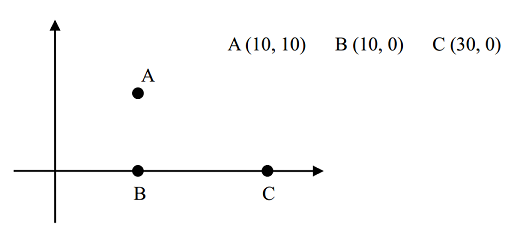【题目描述】【输入】

【输出】

【输入样例】
3 2
10 10
10 0
30 0
【输出样例】
10.00
【提示】


#include <iostream>
#include <cmath>
#include <queue>
#include <cstdio>
#define N 512
#define point pair<int,int>

using namespace std;

struct Edge
{
int from,to;
double dis;
};
int deg;

inline bool operator> (const Edge &a,const Edge &b)
{
return a.dis>b.dis;
}

point list[N];
int n;

int K;

int f[N];

priority_queue <Edge,vector<Edge>,greater<Edge> > Q;

inline double dis(point a,point b)
{
return sqrt((a.first-b.first)(a.first-b.first)+(a.second-b.second)(a.second-b.second));
}

int gz(int k)
{
return f[k]=
(
(
f[k]==k
)    ?
k
:
gz(f[k])
);
}

inline bool hebin(int a,int b)
{
int z1=gz(a),z2=gz(b);
if(z1!=z2)
{
f[z1]=z2;
return true;
}
return false;
}

inline void init()
{

cin.tie(0);
cin>>n>>K;
for(int i=1;i<=n;i++)
{
cin>>list[i].first>>list[i].second;
f[i]=i;
}

for(int i=1;i<=n;i++)
for(int j=1;j<=n;j++)
if(i!=j)
{
Edge t;
t.dis=dis(list[i],list[j]);
t.from=i;
t.to=j;
Q.push(t);
}
}

inline void calc()
{
int cnt=0;
double maxn=0;
while(!Q.empty())
{
Edge t=Q.top();
Q.pop();
if(hebin(t.from,t.to))
{
cnt++;
maxn=max(maxn,t.dis);
}
if(cnt+K>=n)
break;
}
printf("%.2lf",maxn);
}

int main()
{
init();
calc();
}

Last modification：February 7th, 2021 at 06:10 pm